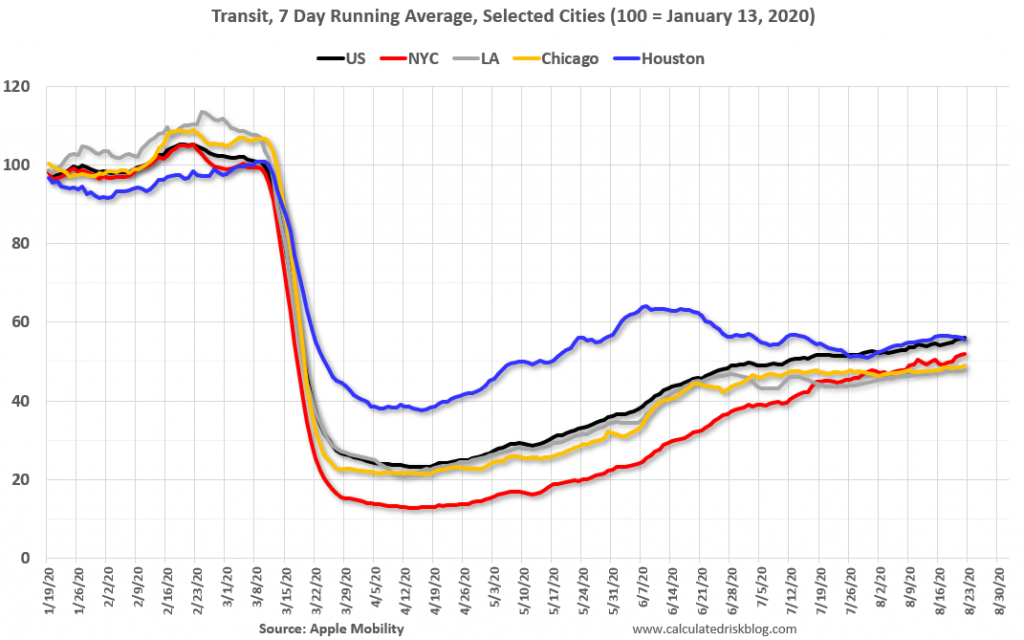Archive | courses

damping covidThis plot, from the Apple mobility site, reminded me of the standard paradigm of linear controlled systems: playing with your gains in linear control systems can affect damping behavior of your system.

It is intuitively clear from the picture, that something separates the NYC, Chicago, LA from Houston: one sees oscillations in Houston, not in the other three metropolises, – so reminiscent of the transition from overdamped to underdamped systems.

What is it? Here’s a toy model which accounts for this phenomenon.…

MATH 199, Monday April 29: Around Arrow

We will be talking about Arrow’s Impossibility theorem, and its topological relatives. The span of the talk will be between American political landscape and elementary algebraic topology – feel free to peruse the links and their vicinity!

Follow-up exercise: try to model your decision process next time you are trying to converge with your friends or relatives, where to go out, or what to watch together. What is the topology of your movie-space?…

Here.…

ECE 515, week of Dec. 3

$$\def\Real{\mathbb{R}}$$

• Pontryagin maximum principle
• Examples
• Lagrangians to Hamiltonians: Fenchel-Legendre transform.

Legendre (sometimes called Legendre-Fenchel) transform $$H$$ of a convex function $$L:\Real\to\Real$$) is defined as
$H(p):=\sup_x px-L(x).$
Both functions, $$L$$ and $$H$$ can take value $$+\infty$$.

If $$L$$ is strictly convex, the gradient mapping
$G:x\mapsto \frac{dL}{dx}$
is one-to-one, and it is clear that the argmax in the definition of $$H$$ solves $$p=G(x)$$. Denote the functional inverse to $$G$$ as $$F: p=G(x) \Leftrightarrow x=F(p)$$. In this case,
$H(p)=pF(p)-L(F(p)). Math 487, week of Dec 3 • Fourier series: smoothness and decay of coefficients. • Fourier transform (following chapter 18 of Zorich, Mathematical Analysis, 2). • Motivation • Examples • Properties • Kotelnikov formula • Asymptotics of integrals (chapter 19 of ibid.) • Laplace method • Localization principle • Standard integrals • Applications: Stirling formula • Tauberian theorems: regularly varying functions Math 487, Homework 5 $$\def\Res{\mathtt{Res}}$$ 1. Find function with Laplace transform equal to \[\frac{s^3 – 1}{(1 + s)^4}.$
2. Find Fourier coefficients of the function $$t^3-\pi^2 t$$ on $$[-\pi,\pi]$$.
Use Parseval’s identity to find $\sum_{n=1}^\infty 1/n^6.$
3. Find general formula for the coefficients of the generating function $$W(z)$$ given by $$W=1+zW^4$$.
4. Find asymptotic frequency of the numbers $$n$$ such that the first digits of $$2^n$$ and $$3^n$$ coincide.

ECE 515, Homework 5

$$\def\Res{\mathtt{Res}}\def\Real{\mathbb{R}}$$

1. Consider optimal control problem for $$x\in\Real, u\in\Real$$:
$\dot{x}=u,\quad J_0^T=\int_0^T\frac{u^2}{2}dt +\cos(x(T))\to\min.$
• 
Find maximal $$T$$ for which the cost function (referred to as $$S$$ in the course notes) at time $$0$$,
$V(x, 0)=inf_{\{u(t)\}_{0\leq t\leq T}, x(0)=x}\ J_0^T$
is smooth as a function of the initial point $$x$$ (i.e. has continuous derivative).

Solution. As we discussed, the cost function for the problem above is given by
$V(x,0)=\min_X C(x,X,T), \mathrm{where} C(x,X,T)=\frac{(x-X)^2}{2T}+\cos(X)$
(here $$X=x(T)$$ is the potential position of the end of the trajectory, and $$(x-X)^2/2T$$ is the minimal cost of reaching it from $$x$$).

Math 487, Nov. 30

• Inverting Fourier transform.
• “Uncertainty principle”.
• Introduction to duality – asymptotics vs. local behavior

ECE 515, Nov. 29

• Kalman identity and some corollaries
• Introduction to the minimum principle

Math 487, Nov. 28

• Fourier series
• Elements of Fourier transform
• Parseval theorem.